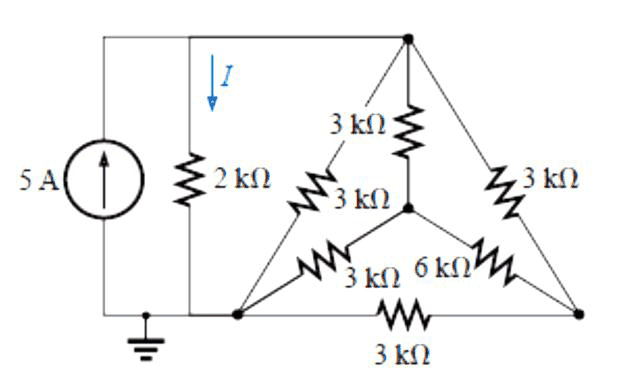# Y-delta/delta-Y transformation problem

yugeci

## Homework StatementThe question asks to find the current I going into the 2k resistor path using Y-delta or delta-Y transformations.

## Homework Equations

Resistance in parallel

1 / R = 1 / R1 + 1 / R2 ..

Converting Delta to Y,

R1 = RaRb / (Ra + Rb + Rc)

Current divider formula

Ix = (Rt / Rx) * Is

## The Attempt at a Solution

I converted the delta network of 3 k Ohm resistors into a Y-network of 1k Ohms each using the above equation. (3*3) / (3 + 3 + 3) = 1. Then I got the total resistance of the network as 1.5 k Ohm. Then I used the current divider formula and found I as 2.14 mA.

The only problem is I'm not sure if I found the total resistance of the network correctly. It's confusing. Can someone tell me if I did it right?

Last edited:

szynkasz
Right, it is ##1.5\,k\Omega##. It is easy to check - delta-Y network is symmetrical so there is no current through ##6\,k\Omega## resistor and you can get rid of it.

Mentor
It looks like you managed to find the correct equivalent resistance of the subnetwork, but I'm not sure how you went about it given that you started by transforming the outer Δ to a Y. The reason I say this is because the "new" Y won't have the same central connection as the existing one, nor will it be at the same potential by symmetry (since the existing one has legs 3-6-3, while the new one has legs 1-1-1 all in kΩ). Just looks like a lot of work from that point!

If you'd done the opposite and transformed the existing Y to a Δ, each new resistor would parallel one in the existing Δ, leaving a single Δ with a "wing" that can collapse to a single resistance and it's easy going from there.

Mentor
Right, it is ##1.5\,k\Omega##. It is easy to check - delta-Y network is symmetrical so there is no current through ##6\,k\Omega## resistor and you can get rid of it.

Ah, so it is! Well spotted!